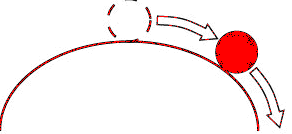## Hooke's law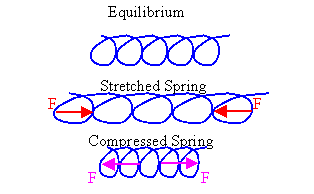A ideal spring has an equilibrium length.  If a spring is compressed, then a force with magnitude proportional to the decrease in length from the equilibrium length is pushing each end away from the other.  If a spring is stretched, then a force with magnitude proportional to the increase in length from the equilibrium length is pulling each end towards the other.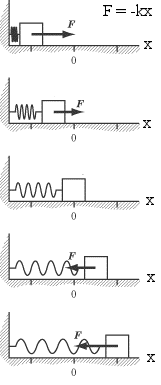The force exerted by a spring on objects attached to its ends is proportional to the spring's change in length away from its equilibrium length and is always directed towards its equilibrium position.

Assume one end of a spring is fixed to a wall or ceiling and an object pulls or pushes on the other end.  The object exerts a force on the spring and the spring exerts a force on the object.  The force F the spring exerts on the object is in a direction opposite to the displacement of the free end.  If the x-axis of a coordinate system is chosen parallel to the spring and the equilibrium position of the free end of the spring is at x = 0, then

F = -kx.

The proportional constant k is called the spring constant.  It is a measure of the spring's stiffness.

When a spring is stretched or compressed, so that its length changes by an amount x from its equilibrium length, then it exerts a force F = -kx in a direction towards its equilibrium position.  The force a spring exerts is a restoring force, it acts to restore the spring to its equilibrium length.

####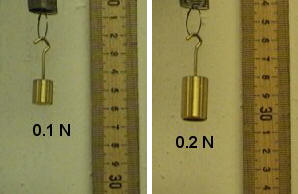Problem:

A stretched spring supports a 0.1 N weight.  Adding another 0.1 N weight, stretches the string by an additional 3.5 cm.  What is the spring constant k of the spring?

Solution:

• Reasoning:
An ideal spring obeys Hooke's law, F = -kx.  The initial stretch is not given.  Let us call it x0.
0.1 N = -kx0. 9.2 N = -k(x0 + 0.035 m).
Subtracting the first from the second equation we have
0.1 N = -k*0.035 m.
• Details of the calculation:
k = |F/x| = (0.1 N)/ (0.035 m) = 2.85 N/m.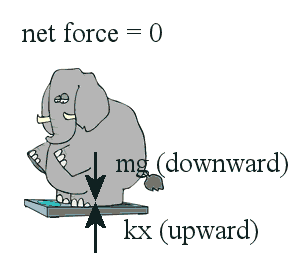You want to know your weight.  You get onto the bathroom scale.  You want to know how much cabbage you are buying in the grocery store.  You put the cabbage onto the scale in the grocery store.

The bathroom scale and the scale in the grocery store are probably spring scales.  They operate on a simple principle.  They measure the stretch or the compression of a spring.  When you stand still on the bathroom scale the total force on you is zero.  Gravity acts on you in the downward direction, and the spring in the scale pushes on you in the upward direction.  The two forces have the same magnitude.

Since the force the spring exerts on you is equal in magnitude to your weight, you exert a force equal to your weight on the spring, compressing it.  The change in length of the spring is proportional to your weight.

Spring scales use a spring of known spring constant and provide a calibrated readout of the amount of stretch or compression.  Spring scales measure forces.  They determine the weight of an object.  On the surface of the earth weight and mass are proportional to each other, w = mg, so the readout can easily be calibrated in units of force (N or lb) or in units of mass (kg).  On the moon, your bathroom spring scale calibrated in units of force would accurately report that your weight has decreased, but your spring scale calibrated in units of mass would inaccurately report that your mass has decreased.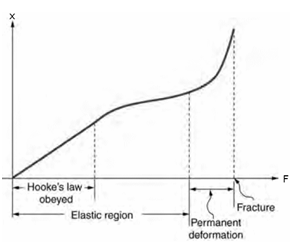Spring scales obey Hooke's law, F = -kx.  Hooke's law is remarkably general.  Almost any object that can be distorted pushes or pulls with a restoring force proportional to the displacement from equilibrium towards the equilibrium position, for very small displacements.  However, when the displacements become large, the elastic limit is reached.  The stiffer the object, the smaller the displacement it can tolerate before the elastic limit is reached.  If you distort an object beyond the elastic limit, you are likely to cause permanent distortion or to break the object.

The elastic properties of linear objects, such as wires, rods, and columns which can be stretched or compressed, can be described by a parameter called the Young's modulus of the material.  Before the elastic limit is reached, Young's modulus Y is the ratio of the force per unit area F/A, called the stress, to the fractional change in length ∆L/L.  (This is an equation relating magnitudes.  All quantities are positive.)

Y = (F/A)/(∆L/L),   F/A = Y∆L/L.

Young's modulus is a property of the material.  It can be used to predict the elongation or compression of an object before the elastic limit is reached.

#### Problem:

Consider a metal bar of initial length L and cross-sectional area A.  The Young's modulus of the material of the bar is Y.  Find the "spring constant" k of such a bar for low values of tensile strain.

Solution:

• Reasoning:
From the definition of Young's modulus:  F = Y A ∆L/L.
From the definition of the spring constant:  F = k∆L.  (Equation, relating magnitudes,  ∆L = magnitude of the displacement from equilibrium.)
Therefore k = Y A/L.

#### Problem:

Consider a steel guitar string of initial length L = 1 m and cross-sectional area A = 0.5 mm2
The Young's modulus of the steel is Y = 2*1011 N/m2
How much would such a string stretch under a tension of 1500 N?

Solution:

• Reasoning:
From the definition of Young's modulus Y = (F/A)/(∆L/L),  we have ∆L = F*L/(Y*A).
• Details of the calculation:
∆L = F*L/(Y*A) = 1500 N*(1 m)/(2*1011 N/m2*0.5 mm2*(1 m/103 mm)2) = 0.015 m = 15 mm.

Consider a point object, i.e. for the moment let us neglect any possible rotation of the object.  If this object is at rest and the net force acting on the object is zero, the object is at an equilibrium position.   If, when slightly disturbed, the object is acted on by a restoring force pointing to its equilibrium position, it is said to be in stable equilibrium.  Objects suspended on springs are in stable equilibrium.  An object sitting on top of a ball, on the other hand, is in unstable equilibrium.  When disturbed, it is acted on by a force pointing away from the equilibrium position.

#### Example:

A ball and a bowl

(a) The ball is in stable equilibrium at the bottom of a bowl.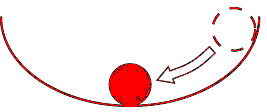(b) The ball is in unstable equilibrium at the top of a bowl.